Students can track their progress and improvement through regular use of ICSE Class 9 Maths Solutions S Chand Chapter 13 Circle Chapter Test.

## S Chand Class 9 ICSE Maths Solutions Chapter 13 Circle Chapter Test

Question 1.
Which type of triangle can be constructed with a 50° angle between two 8 cm sides?
(a) Equilateral
(b) Isosceles
(c) Scalene
(d) Obtuse
Solution:
(b) Isosceles
∵ Two sides are equal and of 8 cm each and their included angle = 50°
∴ The triangle construct with these data will be an isosceles triangle.

Question 2.
Give all names that apply to figure.Solution:
The given figure is a quadrilateral, rectangle parallelogram, rhombus, square.

Question 3.
In the figure, ABCD is a rectanlge. P is the midpiont of $$\overline{AB}$$. Prove ∆DPC is isosceles.Solution:
In the given figure,
ABCD is a rectangle P is mid point of AB.
To prove : ∆DPC is an isosceles triangle
AD = BC (Opposite sides of a rectangle)
AP = PB (P is mid point of AB)
∠A = ∠B (each of 90°)
∴ ∆ADP ≅ ∆BCP (SAS axiom)
∴ DP = CP (c.p.c.t.)
∴ ∆DPC is an isosceles triangle.

Question 4.
In the figure, PQRS is a rhombus with diagonal $$\overline{PR}$$. T is any point on $$\overparen{P R}$$. Prove $$\overparen{\mathrm{TS}}=\overparen{\mathrm{TQ}}$$.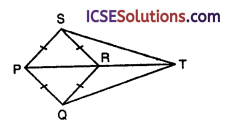Solution:
In the given figure,
PQ = QR = RS = SP
i.e., PQRS is a rhombus with diagonal PR
T is any point on PR produced
ST and QT are joined
To prove : TS = TQ
In rhombus PQRS,
PR is diagonal
∴ ∠PRS = ∠PRQ
But ∠PRS + ∠SRT = 180° (Linear pair)
and ∠PRQ + ∠QRT = 180°
∴ ∠SRT = ∠QRT
Now in ∆SRT and ∆QRT
RT = RT (common)
SR = QR (Sides of rhombus)
∠SRT = ∠QRT (proved)
∴ ∆SRT ≅ ∆QRT (SAS axiom)
∴ ST = QT (c.p.c.t.)
or TS = TQ

Question 5.
In the figure, ABCD is a rhombus. Find :
(i) AB
(ii) m∠ABCSolution:
ABCD is a rhombus whose diagonals bisect each other at right angles and each diagonal bisect opposite angles
(i) BC = CD = AB
⇒ 4x + 15 = 7x + 2
⇒ 7x – 4x = 15 – 2
⇒ 3x = 13
⇒ x = $$\frac { 13 }{ 3 }$$
∴ AB = BC = 4x + 15
= 4 x $$\frac { 13 }{ 3 }$$ + 15 = $$\frac { 52 }{ 3 }$$ + 15
= $$\frac{52+45}{3}=\frac{97}{3}$$ = 32$$\frac { 1 }{ 3 }$$

(b) ∵ Diagonal AC bisect ∠C
∴ ∠BCA = ∠ACD = (4y – 1)°
∴ ∠ADC = 180° – (4y – 1 + 4y – 1)
= 180° – (8y – 2)
∵ Diagonals bisect each other at right angles
∴ ∠AOB = 90° ⇒ 12y = 90°
⇒ y = $$\frac { 90° }{ 12 }$$ = $$\frac { 15 }{ 2 }$$
∴ ADC = 180° – (8 x $$\frac { 15 }{ 2 }$$ – 2)
= 180° – (60° – 2°)
= 180° – 60° + 2°
= 122°Question 6.
Tell whether each statement is sometimes, always, or never true.
(i) A rectangle is a parallelogram.
(ii) A rhombus is a square.
(iii) A parallelogram is a rhombus.
(iv) A rhombus is a rectangle.
(v) A square is a rectangle.
(vi) A rectangle is a quadrilateral.
(vii) A square is a parallelogram.
(viii)A rectangle is a square.
Solution:
(i) A rectangle is a parallelogram It is always.
(ii) A rhombus is a square Sometimes when each angle will be of 90°.
(iii) A parallelogram is a rhombus Sometimes when all sides are equal.
(iv) A rhombus is a rectangle Sometimes, when each angle is of 90° and adjacent sides are unequal.
(v) A square is a rectangle Sometimes when adjacent sides are unequal.
(vi) A rectangle is a quadrilateral Always because a rectangle is one kind of quadrilateral.
(vii) A square is a parallelogram Sometimes when diagonals are unequal and adjacent sides are also unequal.
(viii)A rectangle is a square Sometimes when sides of rectangle are equal.

Question 7.
In the figure, AB = AC, CH = CB and HK is parallel to BC. If the exterior angle CAX is 137°, calculate the angle CHK.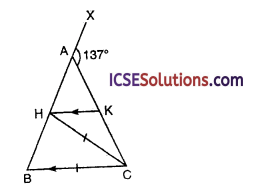Solution:
In the given figure,
AB = AC, CH = CB
HK is parallel to BC
Ext. ∠CAX = 137°
∵ AB = AC
∴ ∠B = ∠C
Ext. ∠XAC = ∠B + ∠C
⇒ 137° = ∠B + ∠B ⇒ 2∠B = 137°
∴ ∠B = $$\frac { 137° }{ 2 }$$ = 68$$\frac { 1° }{ 2 }$$
∵ BC = HC (given)
∴ ∠B = ∠CHB = 68$$\frac { 1° }{ 2 }$$
∴ ∠BCH = 180° – (68$$\frac { 1° }{ 2 }$$ + 68$$\frac { 1° }{ 2 }$$)
= 180° – 137° = 43°
But ∠CHK = ∠BCH (alternate angles)
= 43°

Question 8.
D is a point on the base BC of an isosceles triangle ABC in which AB = AC. The triangle ADE is drawn such that AD = AE, ∠DAE = ∠BAC and D, E are on opposite sides of AC. Prove that
(ii) triangle BAD and CAE are congruent.
(iii) AC bisects ∠BCE.
Solution:
In ∆ABC, AB = AC
D is a point on D and a ∆ADE is drawn
and ∠BAC = ∠DAE
To prove
(iii) AC bisects ∠BCE
Join CE.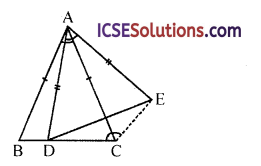Subtracting ∠DAC from both sides
∠BAC – ∠DAC = ∠DAE – ∠DAC

AB = AC (given)
∴ ∆BAD ≅ ∆CAE (SAS axiom)

(iii) ∴ BD = CE
and ∠B = ∠ACE (c.p.c.t.)
But ∠B = ∠ACB (∵ AB = AC)
∴ ∠ACB = ∠ACEQuestion 9.
In the given figure, which is not drawn accurately, ∠BAD = 59°, ∠DAC = 32° and AD = BD. Calculate the value of the angle ACB and state, giving reasons, which is greater, BD or DC.Solution:
In the given figure,
∠BAD = 59°, ∠DAC = 32°
AD = BD To find :
(i) ∠ACB
(ii) Which is greater BD or DC
∴ ∠ABD = ∠BAD = 59°
Now in ∆ABC,
∠A + ∠B + ∠C = 180° (Sum of angles of a triangle)
⇒ (59° + 32°) + 59° + ∠C = 180°
⇒ 91° + 59° + ∠C = 180°
⇒ 150° + ∠C = 180°
⇒ ∠C = 180° – 150° = 30°
Hence ∠ACB = 30°
⇒ DC > BD (∵ AD = BD)
∴ DC is greater

Question 10.
A square ABCD and an equilateral triangle AXB are on the same base AB and on the same side of it. What is the size of the angle CBX? Calculate the angle CXB. The lines AC and BX cut each other at M. Prove that CX is equal to CM.
Solution:
ABCD is a square and ∆ABX is an equilateral triangle.
AC and XC are joined.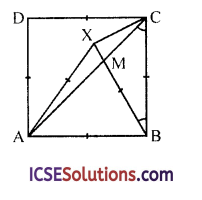In ∆ABC ∠ABX = 60°
(Each angle of an equilateral triangle)
and ∠ABC = 90°
(Each angle of a square)
∴ ∠XBC = ∠ABC – ∠ABX = 90° – 60° = 30°
∵ AC is diagonal
∴ ∠ACB = 45°
In ∆XBC, XC = BC
and ∠XBC = 30°
∴ ∠CXB = ∠XCB = $$\frac{180^{\circ}-30^{\circ}}{2}$$
= $$\frac { 150° }{ 2 }$$ = 75°
and Ext. ∠XMC = ∠MBC + ∠MCB
= 30° + 45° = 75°
∴ ∠CXB = ∠XMC
∴ CX = CM

Question 11.
(i) In the figure, ABCD is an isosceles trapezium. Find the values of x and y.(ii) Given, AC = 5x + 3 and BD = 9x – 12, find the value of x so that ABCD is isosceles.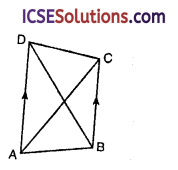(iii) Find SR.Solution:
(i) ABCD is an isosceles trapezium in which AB || DC and AD = BC
∴ 2x + 70° = 180° (Sum of co-interior angles)
⇒ 2x = 180° – 70° = 110°
∴ x = $$\frac { 110° }{ 2 }$$
and ∠D = ∠C ⇒ 2x = y
⇒ 2 x 55° = y ⇒ y = 110°
∴ x = 53°, y = 110°

(ii) In trapezium ABCD which is an isosceles AD || BC
AC = 5x + 3, BD = 9x – 12
∵ ABCD is an isosceles trapezium
∴ AC = BD
⇒ 5x + 3 = 9x – 12
⇒ 9x – 5x = 3 + 12
⇒ 4x = 15 ⇒ x = $$\frac { 15 }{ 4 }$$
∴ x = $$\frac { 15 }{ 4 }$$ = 3.75

(iii) In the given figure,
PQRS is an trapezium in which PQ || SR
M and N are mid points of SP and RQ
MN = 35 and PQ = 40
Let SR = x, then
MN = $$\frac { 1 }{ 2 }$$ (PQ + SR)
⇒ 35 = $$\frac { 1 }{ 2 }$$ (40 + x)
⇒ 40 + x = 35 x 2 = 70
x = 70 – 40 = 30
∴ SR = 30

Question 12.
In the figure, ABCD is a kite whose diagonals intersect at 0. If ∠BAD =110° and ∠BCD = 50°, find
(i) ∠OBC
(ii) ∠ODA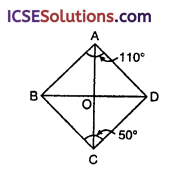Solution:
ABCD is a kite whose diagonals intersect each other at O at right angles
Diagonal AC bisects ∠A and ∠C
∠BAD = 110° and ∠BCD = 50°
(/) ∴ ∠BCO = ∠DCO = $$\frac { 50° }{ 2 }$$ = 25°
In right AOBC, ∠BOC = 90°
∠OBC = 90° – ∠BCO = 90° – 25° = 65°

(ii) Similarly ∠BAO = ∠DAO = $$\frac { 110° }{ 2 }$$ = 55°
∴ In right ∆AOD, ∠AOD = 90°
∴ ∠ODA = 90° – 55° = 35°

Question 13.
Without using set square or protractor, contruct a rhombus in which the diagonals are of length 10 cm and 8 cm and measure one of its sides.
Solution:
Length of diagonals of a rhombus are 10 cm and 8 cm respectively.
Steps of construction :
(i) Draw a line segment AC = 10 cm.
(ii) Draw its perpendicular bisector XY intersecting AC at O.
(iii) Cut off OB = OD = $$\frac { 8 }{ 2 }$$ = 4 cm.
(iv) Join AB, BC, CD and DA.
ABCD is the required rhombus.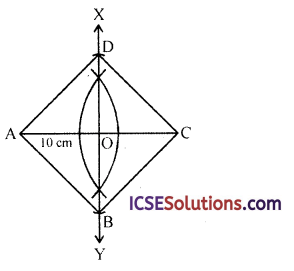Question 14.
Draw parallelogram ABCD with the following data:
AB = 6 cm, AD = 5 cm and ∠DAB = 45°.
Let AC and DB meet in O and let E be the mid-point of BC. Join OE. Prove that
(i) OE || AB
(ii) OE = $$\frac { 1 }{ 2 }$$ AB
Solution:
Steps of construction :
(i) Draw a line segment AB = 6 cm.
(ii) At A, draw a ray AX making an angle of 45° and cut of AD = 5 cm.
(iii) With centre D and radius 6 cm and with centre B, with radius 5 cm, draw arcs intersecting each other at C.
(iv) Join DC and BC.
ABCD is the required parallelogram.
Draw AC and BD intersecting each other at O.
E is the mid-pinot of BC, OE is joined.
In ∆ACB
B and E are the mid points of AC and BC
∴ OE || AB and OE = $$\frac { 1 }{ 2 }$$ AB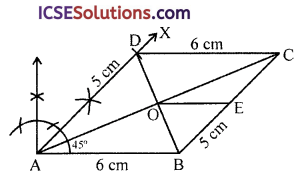Question 15.
In the figure, ABCD is a parallelogram in which angles A and C are obtuse. Points X and Y are taken on the diagonal BD such that the angles XAD and YCB are at right angles. Prove that XA = YC.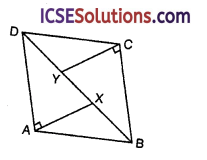Solution:
ABCD is a parallelogram
BD is diagonal and ∠A and ∠C are obtuse
X and Y are points on BD
Such that ∠XAD and ∠YCB are right angle
To prove : XA = YC
AD = BC (opposite sides of parallelogram)
∴ ∆ADX ≅ ∆BCY (ASA axiom)
∴ XA = YC (c.p.c.t.)Question 16.
The sides PQ, PR of a triangle PQR are equal, and S, T are points on PR, PQ such that ∠PSQ and ∠PTR are right angles. Prove that the triangles PTR and PSQ are congruent. If QS and RT intersect at X, prove that the triangles PTX and PSX are congruent.
Solution:
In the given figure, ∆PQR is an isosceles PQ = PR
S and T are points on PR and PQ such that ∠PSQ and ∠PTR are right angles
SQ and TR intersect each other at X
PX is joined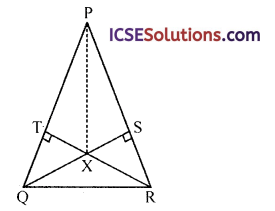To prove :
(i) ∆PTR ≅ ∆PSQ
(ii) ∆PTX ≅ ∆PSX
Proof :
In ∆PTR and ∆PSQ
PR = PQ (given)
∠T = ∠S (each 90°)
∠P = ∠P (common)
∴ ∆PTR ≅ ∆PSQ (AAS axiom)
∴ PT = PS (c.p.c.t.)
Now in ∆PTX and ∆PSX
PX = PX (common)
∠T = ∠S (each 90°)
PT = PS (proved)
∴ ∆PTX ≅ ∆PSX (SAS axiom)

Question 17.
S is the mid-point of the side QR of the triangle PQR, and T is the mid-point of QS. If O is the mid-point of PT, prove that the area of triangle QOT is one-eighth of the area of triangle PQR. ,
Solution:
In the figure in ∆PQR,
S is mid point of QR
T is mid point of QS
O is mid point of PT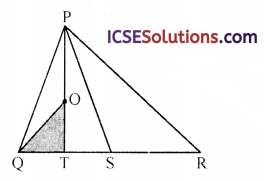To prove : Area ∆OQT = $$\frac { 1 }{ 8 }$$ area ∆PQR
∴ S is mid point of QR
∴ Area ∆PQS = $$\frac { 1 }{ 2 }$$ area ∆PQR
∴ T is mid point of QS
∴ Area ∆PQT = $$\frac { 1 }{ 2 }$$ area ∆PQS
= $$\frac { 1 }{ 2 }$$ x $$\frac { 1 }{ 2 }$$ x area ∆PQR
= $$\frac { 1 }{ 4 }$$ area PQR
∵ O is mid point of PT
area ∆OQT = $$\frac { 1 }{ 2 }$$ area ∆PQT
= $$\frac { 1 }{ 2 }$$ x $$\frac { 1 }{ 4 }$$ area ∆PQR
= $$\frac { 1 }{ 8 }$$ area ∆PQR

Question 18.
In the rhombus PQRS the side PQ = 17 cm and the diagonal PR = 16 cm. Calculate the area of the rhombus.
Solution:
In a rhombus PQRS,
PQ = 17 cm, diagonal PR = 16 cm
Join QS which intersects
PR at O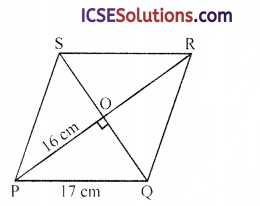∵ Diagonals bisect each other at right angles
∴ PQ = $$\frac { 1 }{ 2 }$$ PR = $$\frac { 16 }{ 2 }$$ = 8 cm
In right ∆POQ
PQ² = PO² + OQ² (Pythagoras Theorem)
⇒ 17² = 8² + OQ²
⇒ 289 = 64 + OQ²
⇒ OQ² = 289 – 64 = 225 = (15)²
∴ OQ = 15 cm
and length of diagonal QS = 2 x OQ
= 2 x 15 = 30 cm
Now area of rhombus = $$\frac { 1 }{ 2 }$$ x product of diagonals
= $$\frac { 1 }{ 2 }$$ x PR x QS
= $$\frac { 1 }{ 2 }$$ x 16 x 30 cm² = 240 cm²Question 19.
In the given figure, not drawn to scale, ∠PSR = 90°, PQ = 10 cm, QS = 6 cm, RQ = 9 cm. Calculate the length of PR.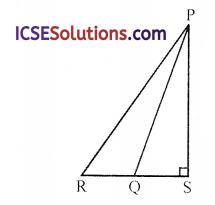Solution:
In the given figure.∠PSR = 90°, PQ = 10 cm, QS = 6 cm
and RQ = 9 cm
To find the length of PR
In right ∆PQS
PQ² = PS² + QS² (Pythagoras Theorem)
10² = PS² – 6² ⇒ 100 = PS² + 36
⇒ PS² = 100 – 36 = 64 = (8)²
∴ PS = 8 cm and RS = 9 + 6 = 15 cm
Now in right APRS
PR² = RS² + PS²
= 15² + 8² = 225 + 64 = 289 = (17)²
PR = 17 cm

Question 20.
In the given figure, AD is the median and DE || AB. Prove that BE is the median.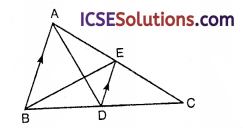Solution:
In the given figure,
DE || BA is drawn which meets AC at E. BE is joined.
To prove : BE is the median In ∆ABC, AD is median
∴ D is mid point of BC
∵ DE || BA
∴ E is mid point of AC
∴ BE is the median

Question 21.
Find:Solution:
(i) In the given-figure,
AD and BE are diameters of the circle with centre O.
∠COD = 90°
m$$\overparen{\mathrm{CDE}}$$
= m$$\overparen{CD}$$ + m$$\overparen{D E}$$
= m$$\overparen{C D}$$ + m$$\overparen{A B}$$ (∠AOB = ∠DOE)
(vertically opposite angles)
= 90° + 18° = 108°

(ii) In the figure, RS and PQ are chords of a circle
m$$\overparen{P Q}$$ = (2x + 27)
and m$$\overparen{R S}$$ = 3x°
∵ RS = PQ
∴ $$m\overparen{\mathrm{PQ}}=m \overparen{\mathrm{RS}}$$
⇒ 2x + 27 = 3x ⇒ 3x – 2x = 27°
⇒ x = 27°
∴ m$$\overparen{R S}$$ = 3x = 3 x 27° = 81°

(iii) In the given figure,
OP is radius and AB is a chord which intersect OP at right angles at Q
and OQ = 5 and QP = 8
Join OB and OA∵ OA = OB = OP = 13 (radius of the circle)
In ∆OQB.
OB² = OQ² + QB²
⇒ 13² = 5² + QB²
⇒ 169 = 25 + QB²
⇒ QB² = 169 – 25 = 144
⇒ QB² = 144 = (12)²
∴ QB = 12 cm
∵ Radius OP is ⊥ to AB
∴ It bisects AB
∴ QB = $$\frac { 1 }{ 2 }$$ AB ⇒ AB = 2QB = 2 x 12 = 24
∴ AB = 24

Question 22.
Determine whether each statement is true or false. If false, explain why.
(i) The central angle of a minor arc is an acute angle.
(ii) Any two points on a circle determine a minor arc and a major arc.
(iii) In a circle, the perpendicular bisector of a chord must pass through the centre of the circle,
Solution:
(i) The central angle of a minor arc is an acute angle.
It is false, because a minor arc < a diameter
∴ Central angle > 180°
Which can be obtuse or right angle.

(ii) Any two points on a circle determine a minor arc and a major arc.
It is false, because the two point can be the ends of a diameter. In this case the arcs are equal.

(iii) In a circle, the perpendicular bisector of a chord must pass through the centre of the circle.
It is true.

Multiple Choice Questions (MCQ’s)

Question 23.
Consider the following statements in respect of any triangle.
I. The three medians of a triangle divide it into six triangles of equal area.
II. The perimeter of a triangle is greater than the sum of the lengths of its three medians. Which of the statements given above is/are correct?
(a) I only
(b) II only
(c) Both I and II
(d) Neither I nor II
Solution:
(c) Both I and II
I. The three medians of a triangle divide it into six triangles of equal area.
II. The perimeter of a triangle is greater than the sum of the lengths of its three medians. Both statements are correct.

Question 24.
The three sides of a triangle are 10, 100 and x. Which one of the following is correct?
(a) 10 < x < 100
(b) 90 < x < 110
(c) 90 ≤ x ≤ 100
(d) 90 ≤ x < 110
Solution:
(b) 90 < x < 110 Three sides of a triangle are 10, 100 and x. We know that sum of any two sides of a triangle is greater than its third. ∴ 10 + x > 100 ⇒ x > 90
or 10 + 100 > x ⇒ x < 110
∴ 90 < x < 110

Question 25.
(i) The median BD of the AABC meets AC at D. if BD = $$\frac { 1 }{ 2 }$$AC, then which one of the following is correct?
(a) ∠ACB = 1 right angle
(b) ∠BAC = 1 right angle
(c) ∠ABC = 1 right angle
(d) None of these
(ii) ABC is an isosceles triangle such that AB = BC = 8 cm and ∠ABC = 90°. What is the length of the perpendicular drawn from Bon AC?
(a) 4 cm
(b) 4$$\sqrt{2}$$ cm
(c) 2$$\sqrt{2}$$ cm
(d) 2 cm
Solution:
(i) The median BD of ∆ABC meets AC at D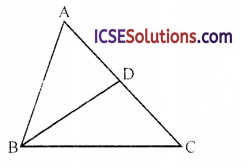If BD = $$\frac { 1 }{ 2 }$$C
BD = $$\frac { 1 }{ 2 }$$ AC = AD = DC
∴ ∠B or ∠ABC = 90°

(ii) ∆ABC is an isosceles triangle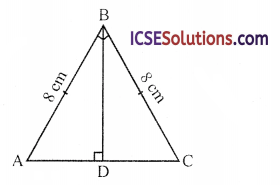AB = BC, ∠ABC = 90°
BD ⊥ AC
In right ∆ABC
AC² = AB² + BC² (Pythagoras Theorem)
= (8)² + (8)² = 64 + 64
= 128 = 64 x 2
∴ AC = $$\sqrt{64 \times 2}$$ = 8$$\sqrt{2}$$ cm
∵ BD ∠AC
BD bisects AC at D
∴ AD = $$\frac { 1 }{ 2 }$$AC = $$\frac { 1 }{ 2 }$$ x 8$$\sqrt{2}$$ = 4$$\sqrt{2}$$
Now in ∆ABD
AB² = BD² + AD² ⇒ 8² = (4$$\sqrt{2}$$)² + BD²
64 = 32 + BD²
⇒ BD² = 64 – 32 = 32 = 16 x 2
∴ BD = $$\sqrt{16×2}$$ = 4$$\sqrt{2}$$ cmQuestion 26.
A ladder 34 m long is placed in a lane so as to reach window 30 m high and on turning the ladder to the other side of the lane and keeping its foot at the same place, reaches a point 16 m high. What is the breadth of the lane?
(a) 18 m
(b) 40 m
(c) 46 m
(d) 50 m
Solution:
In the fiugre. DL or CL is the ladder of length 34 m
On one side of lane it reaches a window 30 m high and on other side of lane, it reaches 16 m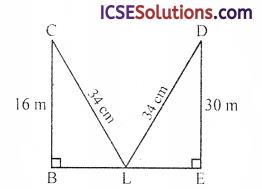In right ∆DEL,
DL² = LE² + DE² (Pythagoras Theorem)
34² = LE² + 30² ⇒ 1156 = LE² + 900
∴ LE² = 1156 – 900 = 256 = (16)²
∴ LE = 16 m
Similarly in right ∆BCL
CL² = BC² + BL² ⇒ 342 = 16² + BL²
⇒ 1156 = 256 + BL² ⇒ BL² = 1156 – 256
∴ BL² = 900 = (30)²
∴ BL = 30 m
Now breadth of the lane = BL + LE
= 30 + 16 = 46 m

Question 27.
In the given figure. ABCD is a square in which AO = AX. What is ∠XOB?(a) 22.5°
(b) 25°
(c) 30°
(d) 45°
Solution:
In a square ABCD diagonal bisect each other at O and AO = AX
In square ∠OAB = $$\frac { 90° }{ 2 }$$ = 45°
In ∆AOX, AO = OX
∴ ∠AOX = ∠AXO
∴ ∠AOX = $$\frac{180^{\circ}-45^{\circ}}{2}$$
= $$\frac{135^{\circ}}{2}=67 \frac{1}{2}^{\circ}$$
But ∠AOB = 90°
(Diagonals of a square bisect each other at right angles)
∴ ∠XOB = ∠AOB – ∠AOX
= 90° – 67$$\frac { 1° }{ 2 }$$
= 22$$\frac { 1° }{ 2 }$$ = 22.5°

Question 28.
In the given figure, AB is a diameter of a circle and CD is perpendicular to AB. If AB = 10 cm and AE = 2 cm, then what is the length of ED?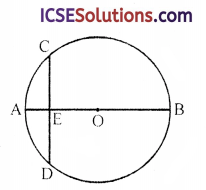(a) 5 cm
(b) 4 cm
(c) $$\sqrt{10}$$cm
(d) $$\sqrt{20}$$cm
Solution:
In the given figure, AB is the diameter of the circle with centre O.
CD ⊥ AB, AB = 10 cm
AE = 2 cm
Join OD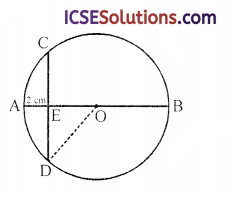AO = $$\frac { 1 }{ 2 }$$ AB = $$\frac { 1 }{ 2 }$$ x 10 = 5 cm
and EO = 5 – 2 = 3 cm
Now in ∆OED
OD² = ED² + EO²
⇒ 5² = ED² + 3²
⇒ ED² = 52 – 32 = 25 – 9
⇒ ED² = 16 = (4)²
∴ ED = 4 cm

Question 29.
In the given figure, AD is a straight line. OP ∠AD and O is the centre of both circles. If OA = 20 cm, OB = 15 cm and OP = 12 cm, what is AB equal to?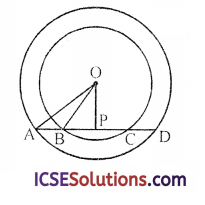(a) 7 cm
(b) 8 cm
(c) 10 cm
(d) 12 cm
Solution:
In the given figure.
AD is a straight line which intersects the concentric circles at A, B, C and D OP ⊥ AD
OA = 20 cm, OB = 15 cm, OP = 12 cm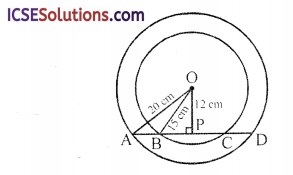In right ∆OAP, by Pythagoras Theorem
OA² = AP² + OP² ⇒ 20² = AP² + 12²
⇒ 400 = AP² + 144
⇒ AP² = 400 – 144
⇒ AP² = 256 = (16)²
∴ AP = 16 cm
Similarly in right ∠OBP
OB² = BP² + OP²
15² = BP² + 12²
⇒ 225 = BP² + 144
⇒ BP² = 225 – 144 = 81 = (9)²
∴ BP = 9 cm
Now AB = AP – BP = 16 – 9 = 7 cm

Question 30.
The length of the common chord of two circles of radii 15 cm and 20 cm whose centres are 25 cm apart is (in cm):
(a) 20
(b) 24
(c) 25
(d) 15
Solution:
Radii of two circles are 15 cm and 20 cm with centres O and C
OC = 25 cm
AB is their common chord
Join OA and CA
AB is perpendicular on OC and OC bisects
AB at D
Join AO and AC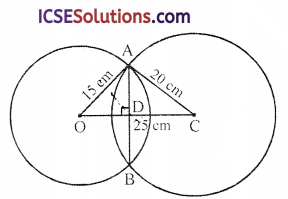Let OD = x. then DC = 25 – x
Now in right ∆AOD
⇒ AD² = 15² – x² = 225 – x² … (i)
Similarly in right ∆ACD
AD² = 20² – (25 – x)² = 400 – 625 + 50x – x² … (ii)
From (i) and (ii),
225 – x² = 400 – 625 + 50x – x²
50x = 225 – 400 + 625 = 850 – 400 = 450
x = $$\frac { 450 }{ 50 }$$ = 9
Now in right ∆AOD
AD² = 225 – 81 = 144 = (12)² cm
and AB = 2 x AD = 2 x 12 = 24 cmQuestion 31.
If a circle with radius 10 cm has two parallel chords 16 cm and 12 cm and they are on the same side of the centre of the circle, then the distance between the two parallel chords is
(a) 5 cm
(b) 8 cm
(c) 2 cm
(d) 3 cm
Solution:
Radius of the circle with centre O = 10 cm Two parallel chord AB and CD are 16 cm and 12 cm
Now we have to find PQ Draw a perpendicular from O to AB and CD intersencting
AB at P and CD at Q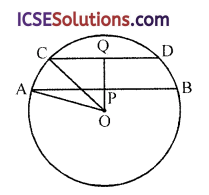∵ The perpendicular bisects the chords at right angles
∴ AP = PB = $$\frac { 16 }{ 2 }$$ = 8 cm
and CQ = QD = $$\frac { 12 }{ 2 }$$ = 6 cm
Join OA and OC
Now in right ∆OAP
OA² = AP² + OP² (Pythagoras Theorem)
⇒ 10² = 8² + OP²
⇒ 100 = 64 + OP²
⇒ OP² = 100 – 64 = 36
⇒ (OP)² = (6)²
∴ OP = 6 cm
Similarly in right ∆OCQ
OC² = CQ² + OQ²
⇒ 10² = 6² + OQ²
⇒ 100 = 36 + OQ²
⇒ OQ² = 100 – 36 = 64 = (8)²
∴ OQ = 8 cm
Now distance between the parallel chords
= OQ – OP = 8 – 6 = 2 cm

Question 32.
In the figure, YZ is parallel to MN, XY is parallel to LM and XZ is parallel to LN. Then MY is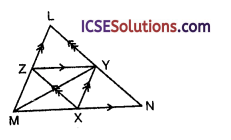(a) the median of ∆LMN
(b) the angular bisector of ∠LMN
(c) perpendicular to LN
(d) perpendicular bisector of LN.
Solution:
In the figure,
YZ || MN, XY || LM and XZ || LN
Then YZ = $$\frac { 1 }{ 2 }$$ MN, XY = $$\frac { 1 }{ 2 }$$ LM and XZ = $$\frac { 1 }{ 2 }$$LN
∴ X, Y and Z are mid points of th sides
∴ MXYZ is a parallelogram
and MY and X∠Are the diagonals of the
parallelogram
∵ Y is mid point of LN
∴ MY is the median of ∆LMN